GRE Subject Test: Physics : Doppler Effect

Example Questions

Example Question #1 : Doppler Effect

A car's horn has a frequency of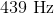. As the car drives away from you, you hear the horn at a frequency of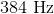. What is the speed of the car?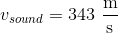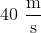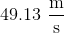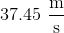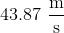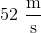Explanation:

The equation for the Doppler effect with frequency for a moving source is given by: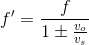Where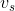is the speed of sound,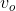is the speed of the source,is the stationary frequency and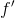is the Doppler frequency. Substituting the known values and solving gives us the speed of the car.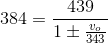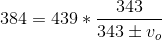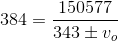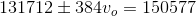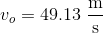All GRE Subject Test: Physics Resources